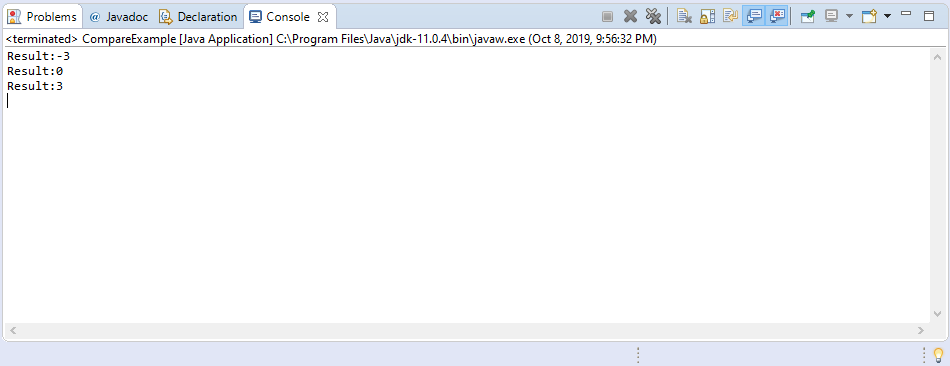Description

The compare​(short x, short y) method of Byte class compares two short values numerically. The value returned is identical to what would be returned by:

Short.valueOf(x).compareTo(Short.valueOf(y))

Method Syntax

public static int compare​(short x, short y)

Method Argument

Data Type Parameter Description
short x the first short to compare
short y the second short to compare

Method Returns

The compare​(short x, short y) method of Short class returns the value 0 if x == y; a value less than 0 if x < y; and a value greater than 0 if x > y.

Compatibility

Requires Java 1.7 and up

Java Short compare​(short x, short y) Example

Below is a simple java example on the usage of compare(short x, short y) method of Short class.

Basically on the above example, we have assigned 3 short primitive x,y,and z. There are 3 examples provided, the first one is when we compare x and y, the second one is x and z, and the last is y and z.

In comparing x and y, the result is -3 which is the difference between x and y. Since the result is less than 0, then we can conclude that x is less than y.

On the second comparison between x and z, the result is 0. As you have already noticed the value of x and z is the same.

For the 3rd example, the comparison is done between y and z. The result would be 3 which is greater than 0. This result means that y is greater than z.

Sample Output

Below is the sample output when you run the above example.